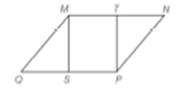Chapter 4.2, Problem 24E### Elementary Geometry for College St...

6th Edition
Daniel C. Alexander + 1 other
ISBN: 9781285195698

#### Solutions

Chapter
Section### Elementary Geometry for College St...

6th Edition
Daniel C. Alexander + 1 other
ISBN: 9781285195698
Textbook Problem
1 views

# In Exercise 19 to 24 , complete each proof. Given: ▱   M N P Q , with T the midpoint of M N ¯ and S the midpoint of Q P ¯ Prove: ∆ Q M S ≅ ∆ N P T and M S P T is a ▱To determine

To prove:

QMSNPT and MSPT is a

Explanation

Given:

MNPQ, with T the midpoint of MN ¯ and S the midpoint of QP¯

 PROOF Statements Reasons 1. ▱MNPQ, with T the midpoint of MN¯ and Sthe midpoint of QP¯ 1. Given 2. MN¯≅PQ¯, andNP¯≅QM¯ 2. Pairs of opposite sides of a parallelogramare congruent 3. ∠MQS≅∠PNT 3

### Still sussing out bartleby?

Check out a sample textbook solution.

See a sample solution

#### The Solution to Your Study Problems

Bartleby provides explanations to thousands of textbook problems written by our experts, many with advanced degrees!

Get Started

#### In Exercises 2336, find the domain of the function. 31. f(x)=xx21

Applied Calculus for the Managerial, Life, and Social Sciences: A Brief Approach

#### Convert the expressions in Exercises 6584 to power form. 3x453x+43xx

Finite Mathematics and Applied Calculus (MindTap Course List)

#### The normal plane to at t = 1 has equation:

Study Guide for Stewart's Multivariable Calculus, 8th